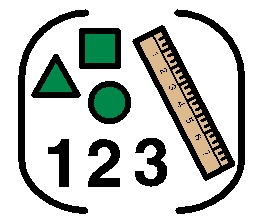# MathsHadrian School uses a modular approach to teach maths. Two week units of structured lessons take place with a specific focus. Lessons are related and enable planning for progression to take place over time, within each unit. Structured Maths lessons are taught on a daily basis.

There are 7 strands;

• Using and applying mathematics
• Counting and understanding number
• Knowing and using number facts
• Calculating
• Understanding shape
• Measuring
• Handling data

There are 2 strands in Maths in the Early Years Foundation Stage

• Numbers
• Shape, space and measure